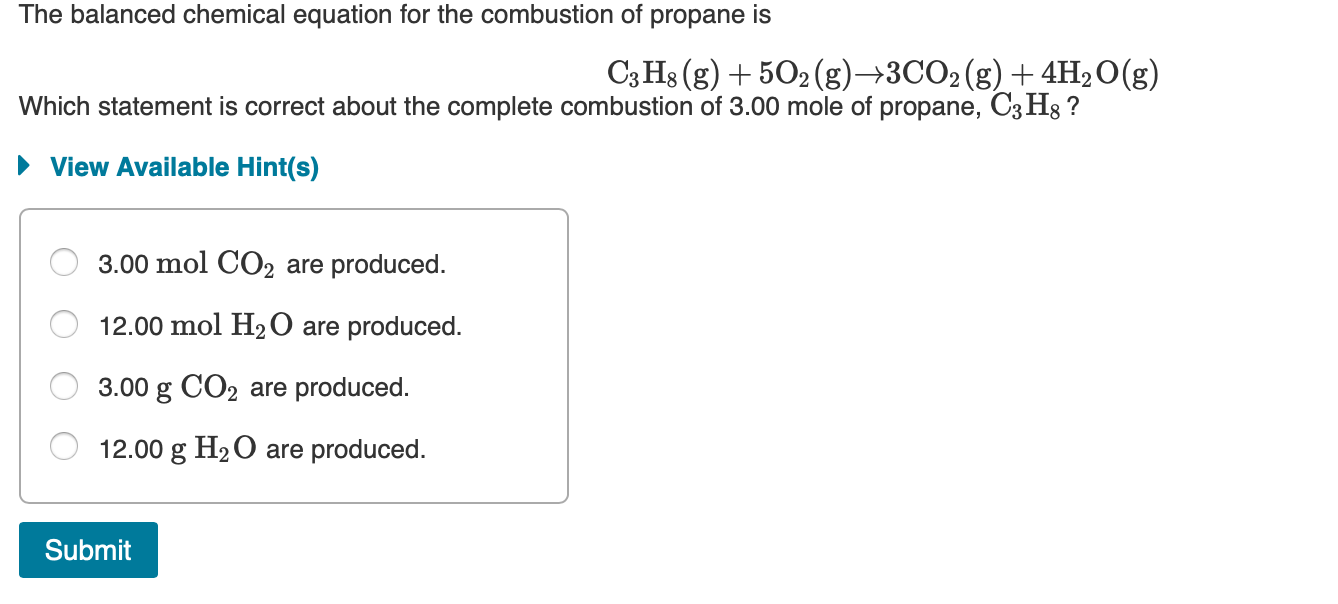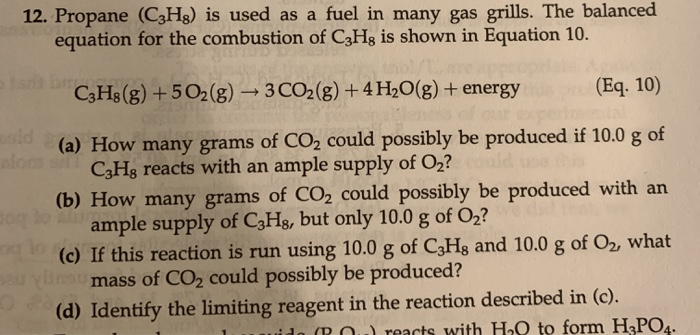QuestionOption B

Since from the balanced equation, 1 mole propane gives 4 mol H2O

Hence 3 mol propane gives 12 mole H2O

#### Earn Coins

Coins can be redeemed for fabulous gifts.

Similar Homework Help Questions
• ### The combustion of propane, C3H8, occurs via the reaction C3H8(g)+5O2(g)→3CO2(g)+4H2O(g) with heat of formation values given...

The combustion of propane, C3H8, occurs via the reaction C3H8(g)+5O2(g)→3CO2(g)+4H2O(g) with heat of formation values given by the following table: Substance ΔH∘f (kJ/mol) C3H8 (g)=  -104.7 CO2(g)= −393.5 H2O(g)= −241.8 Calculate the enthalpy for the combustion of 1 mole of propane.

• ### The combustion of propane (C3H8) produces CO2 and H2O: C3H8 (g) + 5O2 (g) → 3CO2...

The combustion of propane (C3H8) produces CO2 and H2O: C3H8 (g) + 5O2 (g) → 3CO2 (g) + 4H2O (g) The reaction of 7.5 mol of O2 with 1.4 mol of C3H8 will produce ________ mol of CO2. Group of answer choices.

• ### calculate the enthalpy of the combustion of propane for your barbeque C3H8 + 5O2 -> 3CO2 + 4H2O propane -105 kJ...

calculate the enthalpy of the combustion of propane for your barbeque C3H8 + 5O2 -> 3CO2 + 4H2O propane -105 kJ/mol CO2 -394 kJ/mol Water -286 kJ/mol Ooxygen. 0

• ### Consider the combustion of propane: C3H8 (g) + 5O2 (g) → 3CO2 (g) + 4H2O(l) ΔH...

Consider the combustion of propane: C3H8 (g) + 5O2 (g) → 3CO2 (g) + 4H2O(l) ΔH = –2221 kJ Assume that all of the heat comes from the combustion of propane. Calculate ΔH in which 5.00 g of propane is burned in excess oxygen at constant pressure.

• ### ﻿Propane (C3H8)burns according to the following balanced equation: C3H8(g)+5O2(g)→3CO2(g)+4H2O(g) Calculate ΔH∘rxnΔ for this reaction using standard...

﻿Propane (C3H8)burns according to the following balanced equation: C3H8(g)+5O2(g)→3CO2(g)+4H2O(g) Calculate ΔH∘rxnΔ for this reaction using standard enthalpies of formation. (The standard enthalpy of formation of gaseous propane is -103.9 kJ/molkJ/mol.) Express the enthalpy in kilojoules to four significant figures.

• ### C3H8+5O2--->3CO2+4H2O is this chemical equation correct and equal

C3H8+5O2--->3CO2+4H2O is this chemical equation correct and equal? If not why?

• ### The propane fuel (C3H8) used in gas barbeques burns according to this thermochemical equation. C3H8(g)+5O2(g)→3CO2(g)+4H2O(g)ΔH∘rxn=−2044kJ If...

The propane fuel (C3H8) used in gas barbeques burns according to this thermochemical equation. C3H8(g)+5O2(g)→3CO2(g)+4H2O(g)ΔH∘rxn=−2044kJ If a pork roast must absorb 1.7×103 kJ to fully cook, and if only 15 % of the heat produced by the barbeque is actually absorbed by the roast, what mass of CO2 is emitted into the atmosphere during the grilling of the pork roast?

• ### The propane fuel (C3H8) used in gas barbecues burns according to the following equation: C3H8(g)+5O2(g)→3CO2(g)+4H2O(g)ΔH∘rxn=−2044kJ PART...

The propane fuel (C3H8) used in gas barbecues burns according to the following equation: C3H8(g)+5O2(g)→3CO2(g)+4H2O(g)ΔH∘rxn=−2044kJ PART A: If a pork roast must absorb 2.3×103kJ to fully cook, and if only 10.% of the heat produced by the barbecue is actually absorbed by the roast, what mass of CO2 is emitted into the atmosphere during the grilling of the pork roast?

• ### 12. Propane (C3H8) is used as a fuel in many gas grills. The balanced equation for the combustion of C3Hg is shown...12. Propane (C3H8) is used as a fuel in many gas grills. The balanced equation for the combustion of C3Hg is shown in Equation 10. C3H8(g) +502(g) + 3 CO2(g) + 4H2O(g) + energy (Eq. 10) (a) How many grams of CO2 could possibly be produced if 10.0 g of CzHg reacts with an ample supply of Oz? (b) How many grams of CO2 could possibly be produced with an ample supply of C3Hg, but only 10.0 g of O2?...

• ### Given the following equation, C3H8 (g) + 5O2 (g) -> 3CO2 (g) + 4H2O (g); H comb = -2219 kJ/mole A) how much heat will be released when 10 g of propane is burned

Given the following equation, C3H8 (g) + 5O2 (g) -> 3CO2 (g) + 4H2O (g); H comb = -2219 kJ/mole A) how much heat will be released when 10 g of propane is burned? B) how much heat will be released when 10 g of water (H2O) is formed? Please show all work.